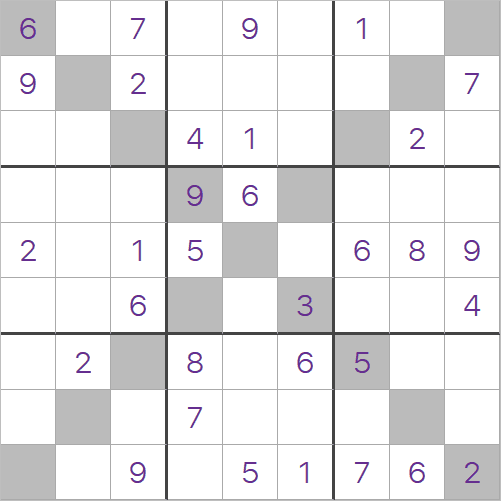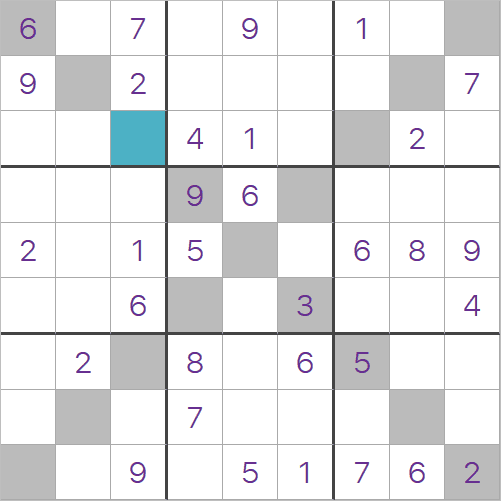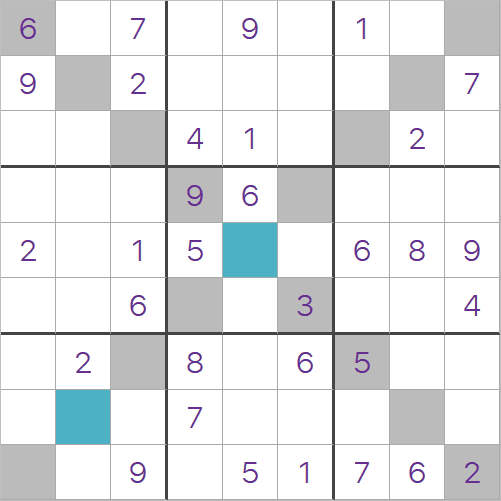X-Sudoku Rules

A brief guideThe rules of X-Sudoku are exactly the same as a normal Sudoku puzzle, but there is one more rule that you need to follow,
• Both the diagonals must contain the numbers 1-9 once and only once.
This is an example of a X-Sudoku puzzle. You can use all the normal techniques you would use to solve a Sudoku puzzle, but you can also take the two diagonals in to account.Look at the highlighted cell in this puzzle. We can eliminate the values 1, 2, 4, 6, 7 and 9 using the normal rules of Sudoku.
If you look at the values already in that diagonal, we eliminate the values 2(again), 3, 5 and 9(again). This just leaves us with the value 8. This cell must be 8!You can also fill in the two highlighted cells. The center cell is on both diagonals, so make sure you consider both diagonals!